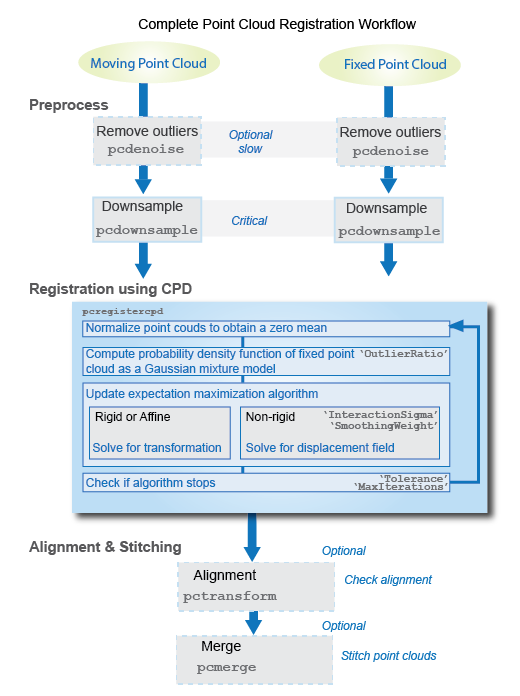# pcregistercpd

Register two point clouds using CPD algorithm

Since R2018b

## Syntax

``tform = pcregistercpd(moving,fixed)``
``[tform,movingReg] = pcregistercpd(___)``
``[___,rmse] = pcregistercpd(___)``
``[___] = pcregistercpd(___,Name=Value)``

## Description

example

````tform = pcregistercpd(moving,fixed)` returns a transformation that registers a moving point cloud with a fixed point cloud using the CPD algorithm. The coherent point drift (CPD) algorithm  supports non-rigid transformations. NoteConsider downsampling point clouds using `pcdownsample` before using pcregistercpd to improve the accuracy and the efficiency of registration. ```
````[tform,movingReg] = pcregistercpd(___)` also returns the transformed point cloud that aligns with the fixed point cloud.```
````[___,rmse] = pcregistercpd(___)` also returns the root mean square error of the Euclidean distance between the aligned point clouds.```
````[___] = pcregistercpd(___,Name=Value)` specifies options using one or more name-value arguments in addition to any combination of arguments from previous syntaxes. For example, `MaxIterations=20` stops the CPD algorithm after 20 iterations.```

## Examples

collapse all

Load point cloud data into the workspace. Extract the moving and the fixed point clouds from the point cloud data in workspace.

```handData = load('hand3d.mat'); moving = handData.moving; fixed = handData.fixed;```

To improve the efficiency and accuracy of the CPD registration algorithm, downsample the moving and the fixed point clouds.

```movingDownsampled = pcdownsample(moving,'gridAverage',0.03); fixedDownsampled = pcdownsample(fixed,'gridAverage',0.03);```

Display the downsampled point clouds before registration.

```figure pcshowpair(movingDownsampled,fixedDownsampled,'MarkerSize',50) xlabel('X') ylabel('Y') zlabel('Z') title('Point clouds before registration') legend({'Moving point cloud','Fixed point cloud'},'TextColor','w') legend('Location','southoutside')```Perform non-rigid registration using the CPD algorithm.

`[tform,movingReg] = pcregistercpd(movingDownsampled,fixedDownsampled);`

Display the downsampled point clouds after registration.

```figure pcshowpair(movingReg,fixedDownsampled,'MarkerSize',50) xlabel('X') ylabel('Y') zlabel('Z') title('Point clouds after registration') legend({'Moving point cloud','Fixed point cloud'},'TextColor','w') legend('Location','southoutside')```## Input Arguments

collapse all

Moving point cloud, specified as a `pointCloud` object.

Fixed point cloud, specified as a `pointCloud` object.

### Name-Value Arguments

Specify optional pairs of arguments as `Name1=Value1,...,NameN=ValueN`, where `Name` is the argument name and `Value` is the corresponding value. Name-value arguments must appear after other arguments, but the order of the pairs does not matter.

Example: `MaxIteration=20` stops the CPD algorithm after 20 iterations.

Before R2021a, use commas to separate each name and value, and enclose `Name` in quotes.

Type of transformation, specified as `"Nonrigid"`, `"Rigid"`, or `"Affine"`.

Data Types: `char` | `string`

Expected percentage of outliers with respect to a normal distribution, specified as a scalar in the range [0, 1). Increasing this value reduces the influence of outliers and noise.

Data Types: `single` | `double`

Maximum number of iterations before CPD stops, specified as a positive integer.

`MaxIterations` and `Tolerance` are both stopping conditions for the CPD algorithm. The algorithm stops when it satisfies either of the stopping conditions.

Data Types: `single` | `double`

Tolerance between consecutive CPD iterations, specified as a scalar. The algorithm stops when the absolute percentage change in the values of the log likelihood function measured between consecutive iterations reaches or falls below the specified tolerance value. Decreasing this value increases the likelihood of a better alignment.

`MaxIterations` and `Tolerance` are both stopping conditions for the CPD algorithm. The algorithm stops when it satisfies either of the stopping conditions.

Data Types: `single` | `double`

Interaction between points, specified as positive scalar that represents standard deviation of a Gaussian filter. Typical values are in the range [1.5, 3]. Increasing this value increases interaction between the points in point cloud. As a result, you can observe coherent motion in the point cloud and every point undergoes the same displacement. Alternatively, decreasing this value reduces interaction between the points in point cloud. As a result, you can observe localized displacement of points and the output displacement field exhibits localized deformation.

Note

To use this name-value argument, `Transform` must be `"Nonrigid"`.

Data Types: `single` | `double`

Motion smoothing weight, specified as a positive scalar. Typical values are in the range [0.1,10]. Increase this value to produce a more coherent motion in the output displacement field.

Note

To use this name-value argument, `Transform` must be `"Nonrigid"`.

Data Types: `single` | `double`

Display progress information, specified as a numeric or logical `0` (`false`) or `1` (`true`). To display the progress information, set `Verbose` to `true`.

## Output Arguments

collapse all

Transformation, returned as a `rigidtform3d` object, an `affinetform3d` object, or a displacement field. `tform` is a 3-D transformation that registers the moving point cloud, `moving` to the fixed point cloud, `fixed`. The output type depends on the value of the `Transform` argument.

`Transform` Value`tform`
`"Rigid"``rigidtform3d` object
`"Affine"``affinetform3d` object
`"Nonrigid"`Displacement field, a numeric matrix of same size and datatype as the `Location` property of the moving point cloud object, `moving`.

Transformed point cloud, returned as a `pointCloud` object. The transformed point cloud is aligned with the fixed point cloud, `fixed`.

Root mean square error, returned as a positive real number. `rmse` is the Euclidean distance between the aligned point clouds.

Data Types: `double`

## AlgorithmsBoth `MaxIterations` and `Tolerance` are used as stopping criteria. The algorithm stops when it satisfies either of the stopping conditions, i.e., when the number of iteration reaches `MaxIterations` or the absolute percentage change in log likelihood function is less than or equal to `Tolerance`.

 Myronenko, A., and X. Song. "Point Set Registration: Coherent Point Drift. "Proceedings of IEEE Transactions on Pattern Analysis and Machine Intelligence (TPAMI)." Vol 32, Number 12, December 2010, pp. 2262–2275.